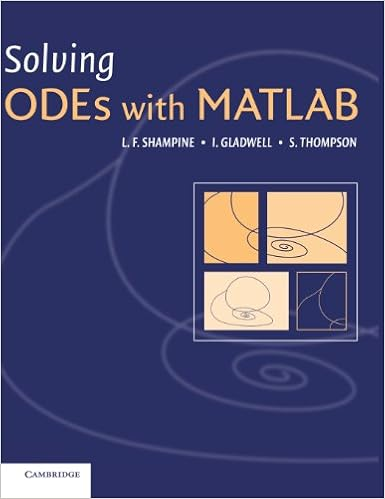## 变分迭代方法解半无限空间常微分方程- 阅读剩余部分 -

## 线性4阶泊松-费米方程的解

4阶泊松-费米方程为：

\begin{equation} \frac{d^2\phi}{dx^2}-\delta_c^2\frac{d^4\phi}{dx^4}=\frac{\sinh \phi}{1+2\gamma \sinh^2 \phi/2}=\rho(\phi) \label{Poisson-Fermi4} \end{equation}

\begin{equation} \delta_c^2\frac{d^4\phi}{dx^4}-\frac{d^2\phi}{dx^2}+\phi=0 \label{LPoisson-Fermi4} \end{equation}

- 阅读剩余部分 -

## matlab 解常微分方程边值问题的程序bvp4c

bvp4c语法

bvp4c实例## 用Matlab bvp4c 解带未知参数的常微分方程边值问题，怎么给出合适的初值？\begin{equation*} \begin{split} f'''-R[(f')^2-ff'']+RA=&0\\ R''+Rfh'+1=&0\\ \theta ''+P_ef\theta '=&0 \end{split} \end{equation*}

\begin{equation*} \begin{split} f(0)=f'(0)=&0\\ f(1)=f'(1)=&1\\ h(0)=h(1)=&0\\ \theta(0)=&0\\ \theta(1)=&1 \end{split} \end{equation*}

- 阅读剩余部分 -

## 用Matlab bvp4c 解带未知参数的常微分方程边值问题\begin{equation*} y''+(100-\beta)y+\gamma y^3=0 \end{equation*}

\begin{equation*} \begin{split} y(-1)=&0\\ y(1)=&0\\ y(-1)=&0.1 \end{split} \end{equation*}

- 阅读剩余部分 -